# HOW TO FIND HYBRIDIZATION OF CENTRAL ATOM & SHAPE OF MOLECULE?

Many students face problems with finding the hybridization of given atom (usually the central one) in a compound and the shape of molecule. Nevertheless, it is very easy to determine the state of hybridization and geometry if we know the number of sigma bonds and lone pairs on the given atom. On this page, I am going to explain you how to determine them in 5 easy steps.

If you are not sure .....What is Hybridization in chemistry?....Watch the following video

## 5 EASY STEPS TO GET THE TYPE OF HYBRIDIZATION & SHAPE

### STEP-1: Write the Lewis structure

It is better to write the Lewis structural formula to get a rough idea about the structure of molecule and bonding pattern. Use the valence concept to arrive at this structure. Concentrate on the electron pairs and other atoms linked directly to the concerned atom.

This step is crucial and one can directly get the state of hybridization and shape by looking at the Lewis structure after practicing with few molecules.

### STEP-2: Calculate the number of sigma (σ) bonds

Number of σ-bonds formed by the atom in a compound is equal to the number of other atoms with which it is directly linked to.

### STEP-3: Calculate the number of lone pairs

The number of lone pairs on a given atom can be calculated by using following formula.v = no. of valence electrons in the concerned atom in free state (i.e. before bond formation).

b = no. of bonds (including both σ & π bonds) formed by concerned atom.

c = charge on the atom (take care: it may not be the charge on entire molecule or ionic species).

Note: When the concerned atom makes a dative bond with other atoms, it may acquire positive or negative charge depending on whether it is donating or accepting the lone pair while doing so respectively.

If it donates a lone pair, a positive charge is accumulated. E.g. Nitrogen atom in ammonium ion, NH4+ gets positive charge since it donates a pair of electrons to H+ ion.

If it receives a lone pair, a negative charge is acquired. E.g. Boron atom gets negative charge when it accepts a lone pair from hydride ion, H- in borohydride ion, BH4-

### STEP-4: Calculate the steric number:

Steric number = no. of σ-bonds + no. of lone pairs

### STEP-5: Assign hybridization and shape of molecule

Now, based on the steric number, it is possible to get the type of hybridization of the atom. Consult the following table.

 Steric number hybridization Structure 2 sp linear 3 sp2 trigonal planar 4 sp3 tetrahedral 5 sp3d trigonal bipyramidal 6 sp3d2 octahedral 7 sp3d3 pentagonal bipyramidal

If the steric number and the number of σ-bonds are equal, then the structure and shape of molecule are same. This case arises when there are no lone pairs on the given central atom.

Note: The structure of a molecule includes both bond pairs and lone pairs. It is always arrived at from the steric number. However, while assigning the shape of molecule, we consider only the spatial arrangement of bond pairs (exclusively of σ-bonds) and atoms connected the central atom.

Hence when the steric number is NOT equal to the number of σ-bonds, we have to arrive at the shape of molecule by considering the arrangement of  the σ-bonds in space. Though the lone pairs affect the bond angles, their positions are not taken into account while doing so.

ILLUSTRATIONS

## WHAT IS THE HYBRIDIZATION OF CARBON IN METHANE, CH4?

### STEP-1: Write the Lewis structure

The valency of carbon is 4 and hence it can form 4 sigma bonds with four hydrogen atoms. Also remember that the valency of hydrogen is one.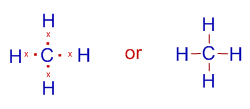### STEP-2: Calculate the number of sigma (σ) bonds

Since carbon is attached to four hydrogen atoms, the number of σ-bonds is equal to 4.

### STEP-3: Calculate the number of lone pairs

The number of lone pairs on carbon atom = (v - b - c) / 2 = (4 - 4 - 0) / 2 = 0.

Note: There are 4 valence electrons in the carbon atom before bond formation.

### STEP-4: Calculate the steric number of carbon atom:

Steric number = no. of σ-bonds + no. of lone pairs = 4 + 0 = 4

### STEP-5: Assign hybridization and shape of molecule

The hybridization of carbon in methane is sp3

This molecule is tetrahedral in structure as well as  in shape, since there are no lone pairs and the number of σ-bonds is equal to the steric number. The bond angle is 19o28'.## DETERMINING THE HYBRIDIZATION OF NITROGEN IN AMMONIA, NH3

### STEP-1: Write the Lewis structure

The valency of nitrogen is 3. Therefore it forms 3 bonds with three hydrogen atoms. There is also a lone pair on nitrogen.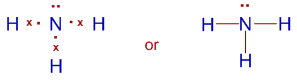### STEP-2: Calculate the number of sigma (σ) bonds

Nitrogen in ammonia is bonded to 3 hydrogen atoms. Hence the number of sigma bonds is equal to 3.

### STEP-3: Calculate the number of lone pairs

The number of lone pairs on nitrogen atom = (v - b - c) / 2 = (5 - 3 - 0) / 2 = 1.

Note: There are 5 valence electrons in the nitrogen atom before the bond formation.

### STEP-4: Calculate the steric number of nitrogen atom:

Steric number = no. of σ-bonds + no. of lone pairs = 3 + 1 = 4

### STEP-5: Assign hybridization and shape of molecule

Nitrogen in ammonia undergoes sp3 hybridization.

The structure of this molecule is based on tetrahedral geometry with one lone pair occupying a corner.

The steric number is not equal to the number of σ-bonds.

Hence the shape is pyramidal (consider only the arrangement of only bonds and atoms in space).

Note: The bond angle is not equal to 109o28'. It is slightly decreased to 107o48' due to repulsion from lone pair.## HYBRIDIZATION AND SHAPE OF AMMONIUM ION, NH4+

### STEP-1: Write the Lewis structure

Let us skip this step.

### STEP-2: Calculate the number of sigma (σ) bonds

The number of sigma bonds formed by nitrogen is 4 since it is bonded to 4 hydrogen atoms.

### STEP-3: Calculate the number of lone pairs

The number of lone pairs on nitrogen atom = (v - b - c) / 2 = (5 - 4 - 1) / 2 = 0.

### STEP-4: Calculate the steric number of central atom:

Steric number = no. of σ-bonds + no. of lone pairs = 4 + 0 = 4

### STEP-5: Assign hybridization and shape of molecule

The hybridization is sp3

Structure is based on tetrahedral geometry.

Shape is also tetrahedral since there are no lone pairs.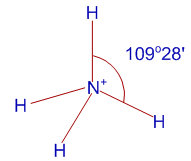## FINDING THE HYBRIDIZATION AND SHAPE OF XeF4

Skip this step.

### STEP-2: Calculate the number of sigma (σ) bonds

The number of sigma bonds formed by xenon is four since it is bonded to only four fluorine atoms.

Note: The valency of fluorine is one.

### STEP-3: Calculate the number of lone pairs

The number of lone pairs on xenon atom = (v - b - c) / 2 = (8 - 4 - 0) / 2 = 2.

Note: Xenon belongs to 18th group (noble gases).

### STEP-4: Calculate the steric number of central atom:

Steric number = no. of σ-bonds + no. of lone pairs = 4 + 2 = 6

### STEP-5: Assign hybridization and shape of molecule

The hybridization is sp3d2

Structure is based on octahedral geometry with two lone pairs occupying two corners.

Shape is square planar.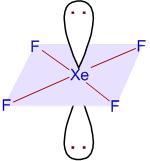Only in above arrangement, the two lone pairs are at 180o of angle to each other to achieve greater minimization of repulsions between them.

If two lone pairs are arranged at 90o of angle, the repulsions are greater. Hence the following structure can be ruled out.## HYBRIDIZATION AND SHAPE OF SULFUR DIOXIDE, SO2

### STEP-1: Write the Lewis structure

Sulfur's valency may be 2 or 4 or 6.

Oxygen's valency is only one.

Hence each oxygen makes two bonds with sulfur atom. Among these, one is sigma bond and the second one is pi bond.

The total number of bonds formed by sulfur with two oxygen atoms is four.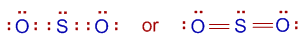### STEP-2: Calculate the number of sigma (σ) bonds

The number of sigma bonds formed by sulfur atom is two since it is bonded to only two oxygen atoms.

### STEP-3: Calculate the number of lone pairs

The number of lone pairs on sulfur atom = (v - b - c) / 2 = (6 - 4 - 0) / 2 = 1.

Number of valence electrons in sulfur is 6. It belongs to 16th group.

Total number of bonds including sigma and pi bonds is 4.

### STEP-4: Calculate the steric number of central atom:

Steric number = no. of σ-bonds + no. of lone pairs = 2 + 1 = 3

### STEP-5: Assign the hybridization and shape of molecule

The hybridization is sp2

Structure is based on trigonal planar geometry with one lone pair occupying a corner.

Shape is angular.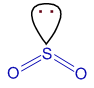< hybridization - illustrations Chemical bonding: TOC